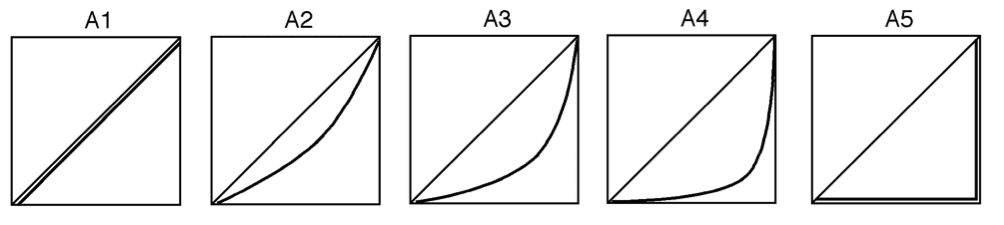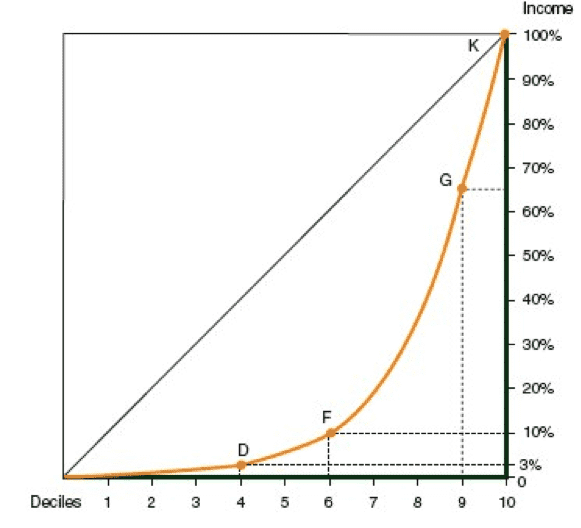# Understanding What is Lorenz Curve

Lorenz Curve

The goal of this curve is to measure the level of income inequality in a country, as compared with other countries.

Becoming familiar with the Lorenz Curve:

The following diagrams display five Lorenz curves, each relating to a different country.   The more concave the curve, then the higher the degree of income inequality in the country. For example:

• Income inequality in Country B is greater than income inequality in Country A.
• Income inequality in Country C is greater than income inequality in Country B.
• There is total income equality in Country A.
• There is total income inequality in Country E, meaning that one household receives all the income of the country, and all other households receive nothing.

Lorenz curves for five countriesSketching the Lorenz Curve

The Lorenz curve represents a concave curve starting at point 0 and ending at point A, as shown in the following diagram.

The symbol used to represent the curve is the letter L.How to Sketch the Lorenz Curve

In this example, the country has 200 households.

Step 1 :

To divide the families into deciles:

a table is prepared, which lists all of the households according to the level of income in descending order.

The list is then divided into 10 groups of equal size (i.e., 20 families each). Each group contains 10% of the country’s households. The top group, which is called the upper decile, contains the wealthiest families in the country, and the bottom group, called the lowest decile, contains the poorest families in the country.

The higher a family’s position on the list, then the greater its income.

Step 2:

Dividing the country income into deciles:

We sum the total income of each decile and calculate its ratio in terms of the combined household income in the country.

Household incomes divided by deciles

 Decile Families Income per household Total income in the decile The decile’s percentage of the total national income Cumulative percentage of the total national income Column 1 Column 2 Column 3 Column 4 Column 5 Column 6 Tenth decile (the upper) 1. The Smiths, 2. The Fields, 3. The Bakers, . . . .,  20. The McDonalds. \$400,00 \$300,00 \$390,000 \$1,750,000 35% 100% Ninth decile 21 – 40 \$1,500,000 30% 65% Eighth decile 41- 60 \$750,000 15% 35% Seventh decile 61 – 80 \$500,000 10% 20% Sixth decile 81 – 100 \$200,000 4% 10% Fifth decile 101 – 120 \$150,000 3% 6% Fourth decile 121 – 140 \$75,000 1.5% 3% Third decile 141 – 160 \$45,000 0.9% 1.5% Second decile 161 – 180 \$25,000 0.5% 0.6% First decile (the lowest) 180 – 200 \$5,000 0.1% 0.1%

Start with the row of the fifth decile. The income of all families in the fifth decile totals \$150,000, amounting to 3% of the country’s total income. If the income of the fifth decile is added to the income of the other deciles below it,

The axes

• The X-axis represents the deciles in ascending order from left to right. The first decile is the poorest, and the tenth decile is the wealthiest.
• The Y-axis represents household income as a percentage of the country’s total income. Percentages rise from bottom to top, i.e., from 0% to 100%, with a space representing 10% between each line.

Every point on the curve corresponds to two numbers.  The diagram displays the Lorenz curve drawn according to the information contained in the table.Explanation of the curve

• Point D shows that the four lowest deciles in the country together earn 3% of the country’s total income (Column 6 of the fourth decile row in Table 6.1). All the other deciles jointly earn the remainder – in this case, 97% of the country’s total income.
• Similarly, point F shows that the six lowest deciles together earn 10% of the country’s entire income. All other deciles, (the top four) earn the remainder – in this case, 90% of the country’s income.
• Point G shows that the nine lowest deciles in the country jointly earn 65% of the total national income. The remaining (top) decile earns the remainder – in this case, 35% of the country’s income.
• Point K shows us that all ten deciles in the country (the entire population) together earn 100% of the country’s entire income.